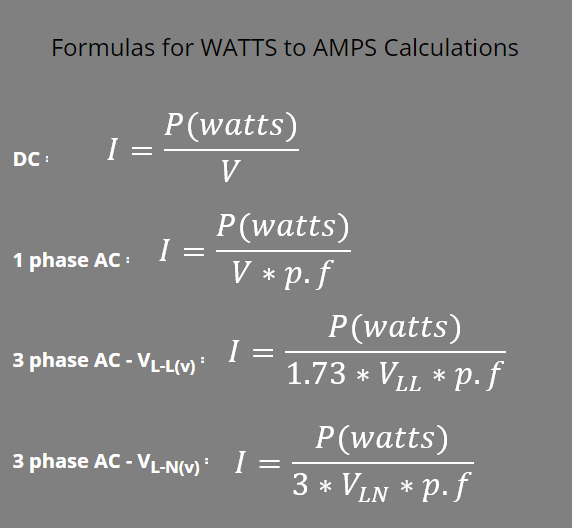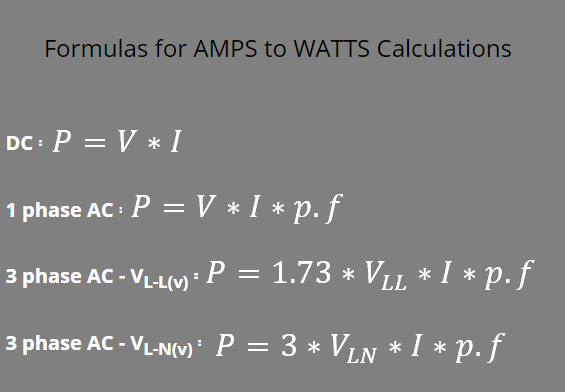(206) 687-4009 | [email protected]

# WATTS TO AMPS AND AMPS TO WATTS CALCULATOR

Watts to amps and amps to watts conversion are useful for designing purpose. For example: for cable and breaker sizing purpose, system power rating (in watts or Kilo watts), voltage and power factor are provided and we convert it into amps through which cable diameter is determine, also its help in fuse and breaker sizing, and deciding starters for different motors.

Choose what to calculate

Connection Type
Power
Voltage
VoltsChoose what to calculate

Connection Type
Current
Amps
Voltage
Volts## What are watts?

Watts are the unit of power. It also expresses the useful electrical energy consumed by a system.

## What is power factor?

Power factor is the ratio of useful energy over supplied energy. For purely resistive system it is 1 and for system having inductance and capacitance it is less than 1 but greater than 0.

For better understanding you can go through this blog.

Power Factor - An Overview

## What is line to line voltage and line to neutral voltage?

A 3 phase system can have two types of connection.

1.Star

2.Delta

In star connection we can have two types of voltage:

• Line to line: voltage between two phases
• Line to neutral:voltage between any of the phase and neutral.

Delta connections have only line to line voltage as no neutral is present in delta connection.

Mostly 3 phase induction motors and generators are connected in star. A user can provide both line to line or line to neutral voltage in order to have line current.

## Watts to Amps or Amps to watts calculator parameter:

• Choose what to calculate:Watts to Amps or Amps to Watts
• if HP to amps selected: rated amps
• If Amps to Watts selected: rated watts. Also user has the option to provide power in watts or Kilowatts (KW).
• Option: For DC, 1∅,, 2∅, and 3∅ circuit
• Rated voltage (volts):for 3 phase voltages, line to line and line to neutral option is provided
• Power factor: in percentage or unit (specified for single phase, two phase and three phase)

## Converting Watts to Amps:

A general step for converting HP to amps for all types of circuit is given as:

Dividing watts with voltage (V) to get the required amps (I).

### For DC circuits:

The formula for DC circuits is given below.

=
P(watts) / V(v)

### For 1 phase AC circuit:

The formula for single phase AC circuit is same as DC with an addition of power factor (p.f) which is given as:

=
P(watts) / PF * V(v)

### For 3 phase AC circuits:

The formula for three phase AC circuit is same as two phase AC circuit but instead of 2 we use square root 3 (~1.73) when voltage is expressed in terms of line to line (VLL) which is given as:

=
P(watts) / 1.73*PF*VLL

When voltage is expressed in terms of line to neutral then we use 3 instead of 1.73.

=
P(watts) 1.733* PF * VL-N(v)

## Converting Amps to Watts:

A general step for converting amps to HP for all types of circuit is given as:

Step1: finding power (in watts) by multiplying voltage(V), current(I) and efficiency (%).

Step2: converting watts to HP, by dividing watts with 746.

### For DC circuits:

The formula for DC circuits is given below.

P =
I(A) * V(V)

### For 1 phase AC circuit:

The formula for single phase AC circuit is same as DC with an addition of power factor (p.f) which is given as:

P =
PF * I(A) * V(V)

### For 3 phase AC circuits:

The formula for three phase AC circuit is same as two phase AC circuit but instead of 2 we use square root 3 (~1.73) when voltage is expressed in terms of line to line (VLL) which is given as:

P =
1.73 * PF * I(A) * VL-L(v)

When voltage is expressed in terms of line to neutral then we use 3 instead of 1.73.

P =
3 * PF * I(A) * VL-N(v)

## Note

In the above formulas:

• As efficiency is expressed in percentage (%) it must be divided by 100 to get the required answer.
• Power factor (p.f) is given in form of unit, ranging from 0 to 1 (for example: 0.8, 0.9). If p.f is expressed in terms of percentage then it is first converted into units by dividing percentage power factor by 100 and then its value is given in the formula.
• Power here in this formula is expressed in watts, if user define it in form of kilowatts then first it is converted into watts by dividing Kilowatts with 1000 and then its value is given in the formula.

## Solved Example:

### For DC circuits:

HP to Amps conversion:

Consider a DC motor that has the following data:

Given:

Voltage =120 V

Power= 300

Required:

Current (I)=? (Amps)

Solution:

From DC circuit formula for HP to Amps conversion:

=
300 / 120
=2.5 Amps

Amps to HP conversion:

Consider a motor that has the following data:

Given:

Voltage =120 V

Current (I)=1.5 Amps

Required:

Power=? (Watts)

Solution:

From DC circuit formula for Amps to HP conversion:

P =
120 * 1.5 /
=180 Watts

### For 1 phase circuits:

HP to Amps conversion:

Consider a single phase AC motor that has the following data:

Given:

Voltage =230 V

Power= 1.5 KW or 1500 W

Power factor (p.f) =0.9

Required:

Current (I)=? (Amps)

Solution:

From single phase AC circuit formula for HP to Amps conversion:

I =
1500 / 230 * 0.9
=7.246 Amps

Amps to HP conversion:

Consider a single phase motor that has the following data:

Given:

Voltage =230 V

Current (I)=10 Amps

Power factor (p.f) =0.9

Required:

Power=? (Watts)

Solution:

From single phase AC circuit formula for Amps to HP conversion:

P =
230 * 10 * 0.9 /
=2070 Watts=2.070 KW

### For 3 phase circuits:

HP to Amps conversion:

Consider a three phase motor that has the following data:

Given:

Voltage (line to line) =480 V

Power= 20 KW or 20,000 W

Power factor (p.f) =0.9

Required:

Current (I)=? (Amps)

Solution:

From three phase AC circuit formula for HP to Amps conversion:

I =
20,000 / 1.73 * 480 * 0.9
=26.761Amps

If we change voltage from line to line to line to neutral, for example: V (line to neutral) =277.13 V

Then we will be calculating it using formula of 3 phase AC circuit when voltage is given as line to neutral that is:

I =
20,000 / 3 * 277.13 * 0.9
=26.729Amps

Amps to HP conversion:

Consider a three phase motor that has the following data:

Given:

Voltage =480 V

Current (I)=25 Amps

Power factor (p.f) =0.9

Required:

Power=? (Watts)

Solution:

From three phase AC circuit formula for Amps to HP conversion:

P =
1.73 * 480 * 25 * 0.9 =18684 Watts=18.684 KW

If we change voltage from line to line to line to neutral, for example: V (line to neutral) =277.13 V (480/1.73)

Then we will be calculating it using formula of 3 phase AC circuit when voltage is given as line to neutral that is:

P =
3 * 277.13 * 25 * 0.9 = 18769.275 Watts= 18.706 KW